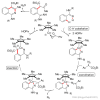# 天行健，君子以自强不息；地势坤，君子以厚德载物。# 天行健，君子以自强不息；地势坤，君子以厚德载物。

Come on and fight so that you will come into sky.

### CSP2020 J/S 游记

posted on 2020-11-08 20:04:30 | under OI 生活记录 |

## 提高复赛

$T_2,T_3$ 直接上暴力骗分。

$T_4$ 跟 $T_1$ 情况差不多，就连错误都是一样，有些输出刚刚好，有些比答案大了 $1$……

## 提高考完

KFC 吃晚餐，打包上车，回贫困山区！

## 洛谷估分

• 普及：$100+100+30+100=330$
• 提高：$40+0+15+5=60$（我是蒟蒻）

## 考试成绩

• 普及：$100+100+35+100=335$（其实 $T_3$ 再认真思考思考可以 $\texttt{AK}$ 的啊）
• 提高：$40+40+10+5=95$（我是蒟蒻，这个成绩耻辱啊）

## 对自己的一些话

$2020$ 年可能是最后一次去考普及了，还有 $2$ 年的 OI 龄就要退役了，时光飞逝啊，加油吧，既然第一次提高已经失利，那么就认认真真地为下一年的提高准备吧。